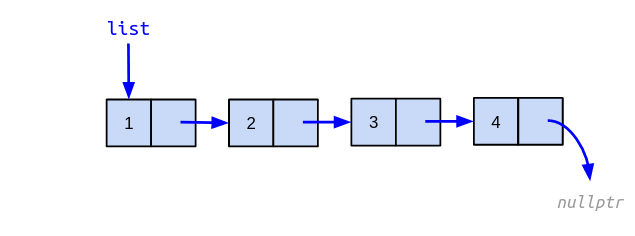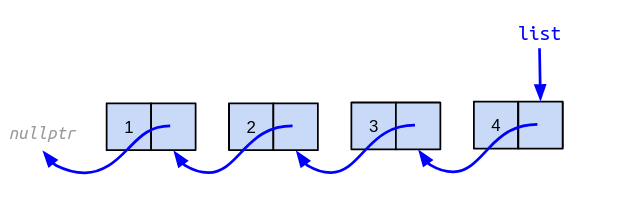# Overview: Final Exam

## Topics

1. Algorithm Basics. [STEPHENS] chapter 1.
1. Basic concepts (algorithms, data structures, pseudocode).
2. Big O notation.
3. Common runtime functions: $$O(1)$$, $$O(\log N)$$, $$O(N)$$, $$O(N \log N)$$, $$O(N^2)$$, $$O(N^3)$$, $$O(2^N)$$, $$O(N!)$$. Examples of algorithms for each of these.
2. Memory Management in C++
1. Correct usage of dynamic memory operators: new and delete for simple objects and arrays.
2. Implementation of class destructors.
3. Diagnosing and correcting memory errors (segmentation faults and memory leaks) using Valgrind.
3. Linked Lists. [STEPHENS] chapter 3.
3. Circular lists.
4. Node insertion and deletion.
5. Sequential traversal.
6. Using sentinels.
4. Stacks and Queues. [STEPHENS] chapter 5.
1. LIFO and FIFO methods.
2. Inserting and removing elements.
3. Implementions using linked lists, arrays, and circular arrays.
4. Evaluation of expressions in postfix notation.
5. Common examples.
5. C++ Standard Containers, Part I (simple usage and basic operations).
1. std::list
2. std::forward_list
3. std::vector
4. std::array
5. std::stack
6. std::queue
6. Sorting. [STEPHENS] chapter 6.
1. $$O(N^2)$$ algorithms (insertiosort, selectionsort, bubblesort).
2. $$O(N \log N)$$ algorithms (heapsort, quicksort, mergesort).
3. Sub $$O(N \log N)$$ algorithms (countingsort, bucketsort).
4. C++ std::sort function using a custom comparator.
7. Searching. [STEPHENS] chapter 7.
1. Linear search.
2. Binary search.
8. Hash Tables. [STEPHENS] chapter 8.
1. Hashing functions.
2. Collisions.
3. Chaining.
4. Open addressing and linear probing.
9. Recursion. [STEPHENS] chapter 9.
1. Design and implementation of recursive algorithms.
2. Base case and recursive step.
3. Tracing recursive function execution using droid diagrams.
4. Common examples.
10. Binary Trees. [STEPHENS] chapter 10.
1. Tree terminology and properties (ancestor, binary tree, binary search tree, branch, child, complete tree, degree, descendant, full tree, height, internal node, leaf node, level, parent, perfect tree, root, sibling, subtree).
2. Searching and node insertion in a binary search tree.
3. Tree traversal algorithms (inorder, preorder, postorder, levelorder).
4. Design and implementation of tree operations.
5. Expression trees.
11. C++ Standard Containers, Part II (simple usage and basic operations).
1. std::set
2. std::map
3. std::unordered_set
4. std::unordered_map

## Allowed Items During the Exam

NOTE: Once the exam starts you are not allowed to share any items with anyone else.

1. Pen, pencil, eraser, pencil sharpener.

2. Simple scientific calculator. You are not allowed to use a cell phone, programmable calculator, tablet, computer, or any other electronic device.

3. Personal cheat sheet with the following characteristics:

 Must be one of these: $$5 \times 8$$ inches index card ($$12.7 \times 20.32$$ cm). Half letter size sheet of paper ($$13.97 \times 21.59$$ cm). Must be handwritten. Computer-made cards/sheets are not allowed. You can write in both sides of the card/sheet. Must include your student ID and full name on the upper-left corner of both sides of the card/sheet. There are no restrictions on the specific content written on the card/sheet.

## Sample Questions

1. Name an algorithm that has an $$O(\log N)$$ complexity.
2. You have three algorithms: A, B, and C. Algorithm A has a performance of $$O(N!)$$. Algorithm B has a performance of $$O(N^3)$$. Algorithm C has a performance of $$O(2^N)$$. Which algorithm has the best performance? Which one has the worst?

3. You have the following C++ code:

#include <iostream>

int f(int n)
{
int r = 1;
for (int i = 0; i < n; ++i) {
r *= 2;
}
return r;
}

void g(int n)
{
int r = f(n);
for (int i = 0; i < r; ++i) {
std::cout << i << " ";
}
std::cout << "\n";
}


What is the time complexity of function g? Explain your answer.

4. Assume that the following code completes the program that started with the code from the previous question. What gets printed to the standard output when running the program?

int main()
{
g(5);
return 0;
}

5. When trying to find a certain item inside a linked list, which search algorithm should be used and what is its time complexity?

6. Assume you have the following C++ struct declaration:

struct Node {
int value;
Node* next;
};


Using this structure it is possible to create a singly linked list using dynamic memory. For example:Write a reverse function that inverts a linked list by changing the links between nodes. No new nodes should be created. The value field of the nodes should not be modified. For the list shown above, the resulting inverted list should be as follows:The signature of the reverse function should be:

void reverse(Node*& list)

7. What gets printed to the standard output when running the following C++ code?

#include <iostream>
#include <queue>
#include <stack>

int main()
{
std::stack<int> s;
std::queue<int> q;
q.push(4);
q.push(8);
q.push(15);
q.push(16);
q.push(23);
q.push(42);
while (not q.empty()) {
int x = q.front();
q.pop();
if (x % 2 == 0) {
s.push(x);
}
}
while (not s.empty()) {
int x = s.top();
s.pop();
std::cout << x << " ";
}
std::cout << "\n";
return 0;
}

8. Given the following postfix expression:

$$3 \; 5 \; 6 \; + \; * \; 10 \; 7 \; - \; - \; 3 \; /$$
1. Obtain the result of its evaluation.
2. Build its corresponding expression tree.
3. Provide its equivalent infix expression (use parenthesis to avoid incorrect operator precedence).
9. What gets printed to the standard output when running the following C++ code?

#include <algorithm>
#include <iostream>
#include <vector>

bool comparator(int a, int b)
{
return std::abs(a) <= std::abs(b);
}

int main()
{
std::vector<int> v {-35, 12, -43, 50, -77, -76, -8, 69};
std::sort(v.begin(), v.end(), comparator);
for (int x : v) {
std::cout << x << " ";
}
std::cout << "\n";
return 0;
}

10. This sorting algorithm uses the fairly obvious fact that, if an array is not sorted, it must contain two adjacent elements that are out of order. The algorithm repeatedly passes through the array, swapping items that are out of order, until it can't find any more swaps. What’s the name of this algorithm?

11. You have the following hash function:

$$H(k) = k * 97 + 5$$

Given a hash table implemented using open addressing and linear probing on an integer array of size 15, indicate the final state of that table after inserting the following numbers using the hash function above:

$$54, 45, 92, 22, 15, 90$$
12. In order to solve the Tower of Hanoi puzzle with ten disks, at least how many moves do you need?

13. Build a binary search tree by inserting the following values:

$$34, 15, 25, 40, 60, 51, 9, 31, 87, 71, 24$$

Traverse the resulting tree in inorder, preorder, postorder, and levelorder.

14. You are given a binary tree with the following traversals:

• Inorder: B A F D G C H E
• Preorder: A B C D F G E H

With this information rebuild the corresponding binary tree.

15. You have a perfect binary tree with 511 nodes. What is the height of this tree?

16. Draw four different binary trees of height $$H=2$$ that are also:

1. A perfect tree.
2. A complete tree that is not full.
3. A full tree that is not complete.
4. A tree with the least amount of nodes.

17. You have the following struct declaration that represents a binary tree node.

struct Node {
Node* left;
Node* right;
};


Write in C++ or pseudocode a recursive function called node_count that returns the total number of nodes contained in a given tree. The function’s signature is as follows:

int node_count(Node* p)

18. What’s the output of the following C++ program?

#include <iostream>
#include <map>
#include <vector>

int main()
{
std::map<int, std::vector<int>> m;
for (int n = 1; n <= 10; ++n) {
std::vector<int> v;
for (int i = 1; i <= n; ++i) {
if (n % i == 0) {
v.push_back(i);
}
}
m[n] = v;
}
for (auto p : m) {
std::cout << p.first << ": ";
for (int x : p.second) {
std::cout << x << " ";
}
std::cout << "\n";
}
return 0;
}